Theory of Machines

# Theory of Machines

Test Description

## 25 Questions MCQ Test GATE Mechanical (ME) 2023 Mock Test Series | Theory of Machines

Theory of Machines for Mechanical Engineering 2023 is part of GATE Mechanical (ME) 2023 Mock Test Series preparation. The Theory of Machines questions and answers have been prepared according to the Mechanical Engineering exam syllabus.The Theory of Machines MCQs are made for Mechanical Engineering 2023 Exam. Find important definitions, questions, notes, meanings, examples, exercises, MCQs and online tests for Theory of Machines below.
Solutions of Theory of Machines questions in English are available as part of our GATE Mechanical (ME) 2023 Mock Test Series for Mechanical Engineering & Theory of Machines solutions in Hindi for GATE Mechanical (ME) 2023 Mock Test Series course. Download more important topics, notes, lectures and mock test series for Mechanical Engineering Exam by signing up for free. Attempt Theory of Machines | 25 questions in 75 minutes | Mock test for Mechanical Engineering preparation | Free important questions MCQ to study GATE Mechanical (ME) 2023 Mock Test Series for Mechanical Engineering Exam | Download free PDF with solutions
 1 Crore+ students have signed up on EduRev. Have you?
*Answer can only contain numeric values
Theory of Machines - Question 1

### If the ratio of maximum and minimum speeds of a flywheel is 1.11 and the kinetic energy at mean speed is 250 kJ. Maximum fluctuation of energy is ___________ kJ (Important - Enter only the numerical value in the answer)

Detailed Solution for Theory of Machines - Question 1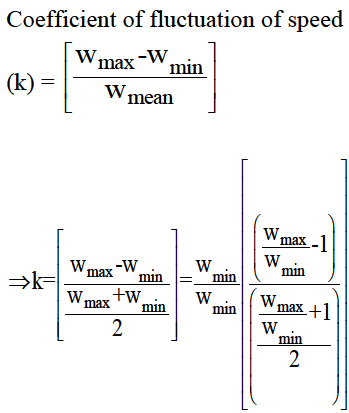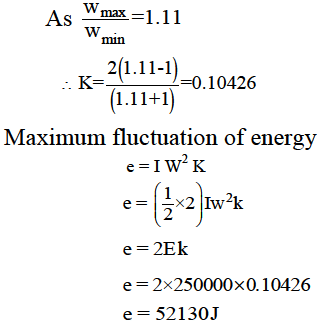e = 52.13kJ

*Answer can only contain numeric values
Theory of Machines - Question 2

### The moment of inertia of flywheel is 2000 kgm2 starting from rest it is moving with a uniform acceleration of 0.5 radian/sec2, After 10 sec from start, its kinetic energy will be ______ Nm (Important - Enter only the numerical value in the answer)

Detailed Solution for Theory of Machines - Question 2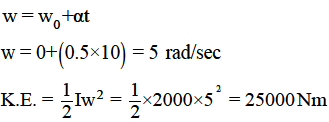Theory of Machines - Question 3

### Calculate the thrust in connecting rod, if piston effort is 200 kN and crank makes an angle of 45o from TDC. Assume obliquity ratio = 3.5

*Answer can only contain numeric values
Theory of Machines - Question 4

A machine is coupled to a two stroke engine which produces a torque of [800 + 180 sin 3θ] [N m], where θ is the crank angle. The maximum fluctuation of energy, if resisting torque is constant is ___________ Nm

(Important - Enter only the numerical value in the answer)

Detailed Solution for Theory of Machines - Question 4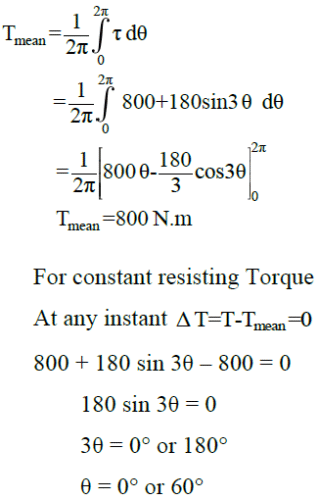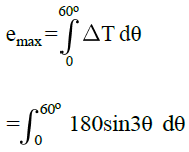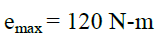*Answer can only contain numeric values
Theory of Machines - Question 5

When bar AB is in the position shown, end B is sliding to the right with a velocity of 0.8 m/ sec. Velocity of A in this position is –––––– m/sec.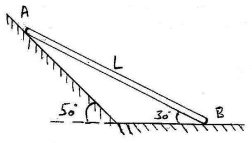(Important - Enter only the numerical value in the answer)

Detailed Solution for Theory of Machines - Question 5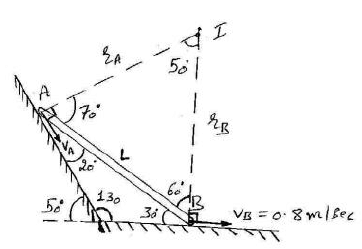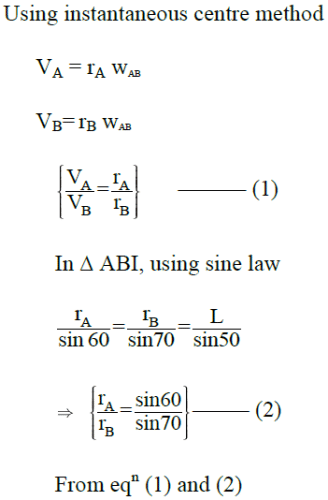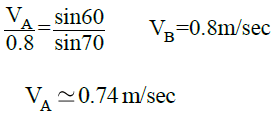Theory of Machines - Question 6

A rod AB experiences different velocities at point A and B. Figure shows the instant when the rod is horizontal while moving in the vertical plane. At this instant the location of instantaneous centre for velocities and the angular velocity of the rod respectively are.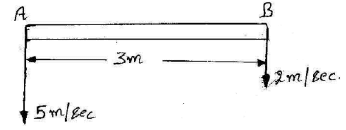Detailed Solution for Theory of Machines - Question 6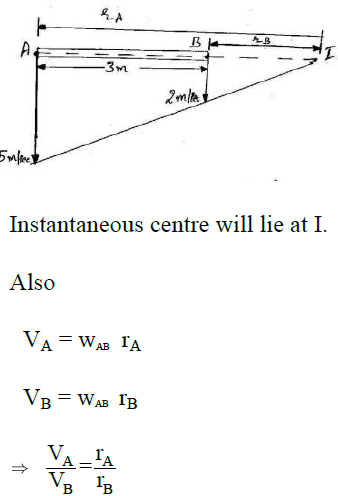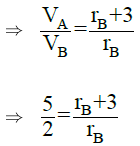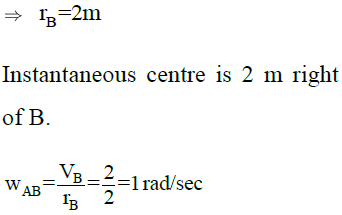*Answer can only contain numeric values
Theory of Machines - Question 7

A riveting machine is driven by a motor of 3 KW. It takes 1 sec and 12 KN-m of energy to complete one riveting operation. The moment of Inertia of fly wheel is 55 (kg m2). The speed of the fly wheel immediately after riveting, if it is 360 rpm before riveting is ––––––– rpm.

(Important - Enter only the numerical value in the answer)

Detailed Solution for Theory of Machines - Question 7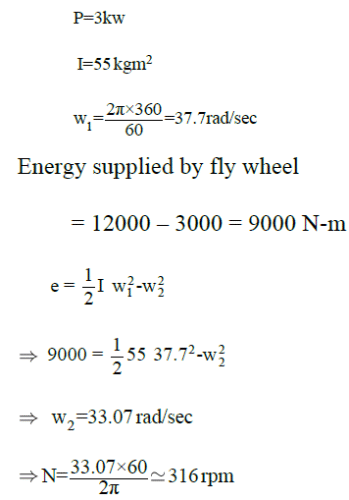*Answer can only contain numeric values
Theory of Machines - Question 8

In a certain slider crank mechanism length of connecting rod is very large as compared to crank length. If x is the displacement of piston at the moment when the crank has turned through an angle of 30° from Inner dead centre, for crank length of 300 mm, value of x = –––– mm.

(Important - Enter only the numerical value in the answer)

Detailed Solution for Theory of Machines - Question 8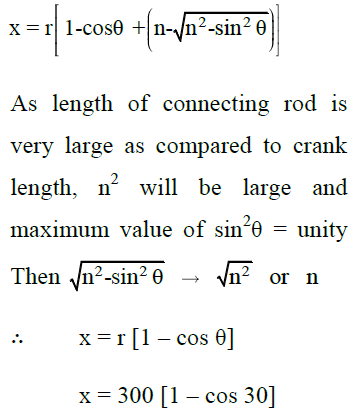Theory of Machines - Question 9

In a slider crank mechanism, the maximum acceleration of slider is obtained when the crank is

Detailed Solution for Theory of Machines - Question 9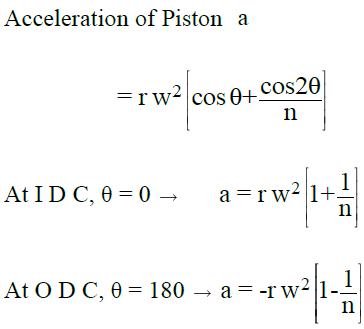Theory of Machines - Question 10

In the case of fly wheel, the maximum fluctuation of energy is.

Detailed Solution for Theory of Machines - Question 10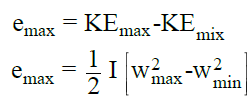Theory of Machines - Question 11

In the diagram given, the magnitude of absolute angular velocity of link 2 is (10 rad/sec) while that of link 3 is (6 rad/sec). Angular velocity of link 3 relative to 2 is –––––––– rad/sec. [Magnitude only]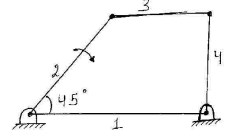(Important - Enter only the numerical value in the answer)

Detailed Solution for Theory of Machines - Question 11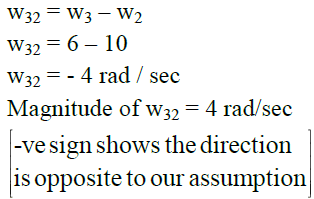Theory of Machines - Question 12

In an elliptical trammel, the length of the link connecting the two sliders is 100 mm. The tracing pen is placed on 150 mm extension of this link. The major and minor axes of the ellipse traced by the mechanism would be

Detailed Solution for Theory of Machines - Question 12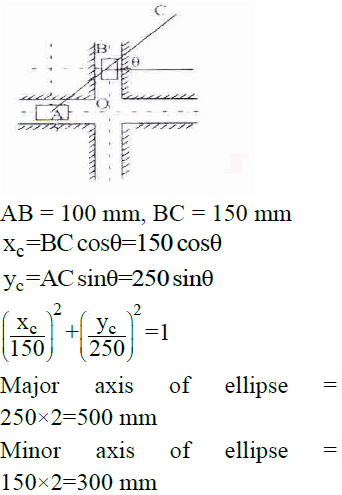*Answer can only contain numeric values
Theory of Machines - Question 13

Degrees of freedom of following mechanism is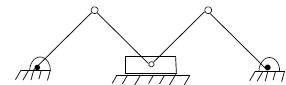(Important - Enter only the numerical value in the answer)

Detailed Solution for Theory of Machines - Question 13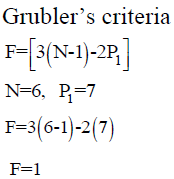Theory of Machines - Question 14

In a bar mechanism with all four links of different lengths, doublecrank behavior was observed when one of the links was fixed. After modification if the fixed links is set free and the link adjacent to it is fixed. The mechanism will behave as:-

Detailed Solution for Theory of Machines - Question 14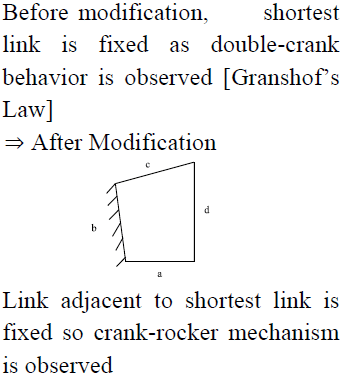Theory of Machines - Question 15

The given figure shows a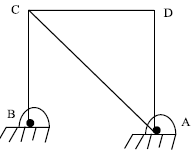Detailed Solution for Theory of Machines - Question 15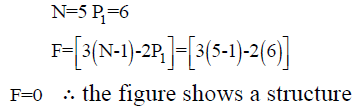Theory of Machines - Question 16

At an instant a circular body P is observed [refer figure]. Velocities at point A and B are horizontal and opposite in direction. The instantaneous centre of rotation of the body will lie at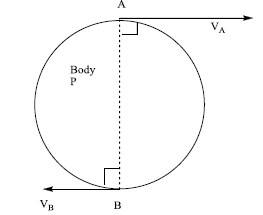Detailed Solution for Theory of Machines - Question 16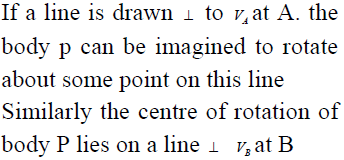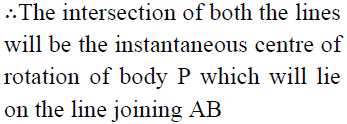Theory of Machines - Question 17

No. of Ternary links and degree of freedom of the mechanism respectively are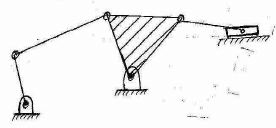Detailed Solution for Theory of Machines - Question 17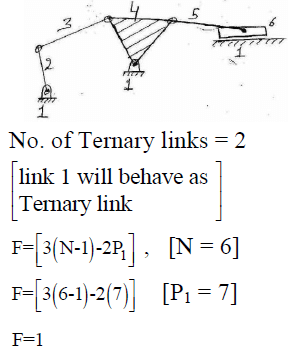*Answer can only contain numeric values
Theory of Machines - Question 18

In the mechanism shown, the degree of freedom using Gruebler’s criterion is ________ rad/s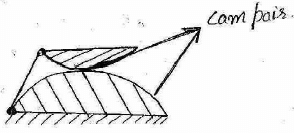(Important - Enter only the numerical value in the answer)

Detailed Solution for Theory of Machines - Question 18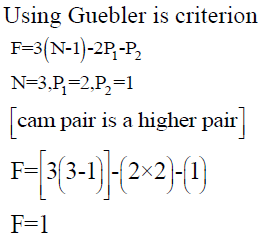*Answer can only contain numeric values
Theory of Machines - Question 19

In the given figure the mechanism has single degree of freedom. At an instant when the link 3 makes an angle of 600 with horizontal, the downward velocity of slider link 2 is 1 m/sec. if the length of link 3 is 1 m. The angular velocity of link 3 at this instant will be_______ (rad/sec)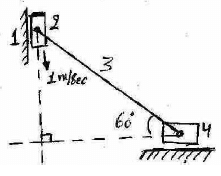(Important - Enter only the numerical value in the answer)

Detailed Solution for Theory of Machines - Question 19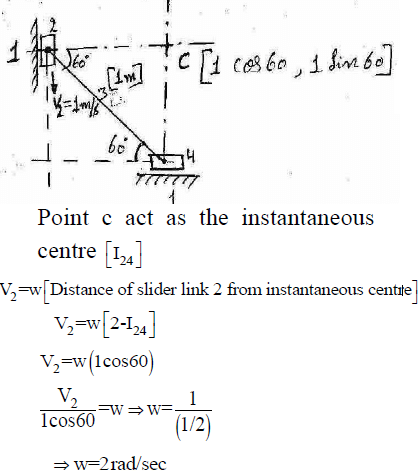Theory of Machines - Question 20

To use slider-crank chain mechanism in shaper to have a quick return stroke, which of the links is to be fixed?

Detailed Solution for Theory of Machines - Question 20

When shortest or crank link is fixed, Whitworth quick return motion mechanism is obtained which is used in shaper

*Answer can only contain numeric values
Theory of Machines - Question 21

Figure shows a wheel rotating about centre O. Two points A and B located on the diameter O1 O2 of the wheel have speeds of 90 m/sec and 105 m/sec respectively. Distance b/w points A and B is 650 mm. diameter of the wheel is _____ mm
[BO2 is 50 mm]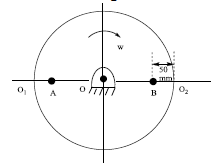(Important - Enter only the numerical value in the answer)

Detailed Solution for Theory of Machines - Question 21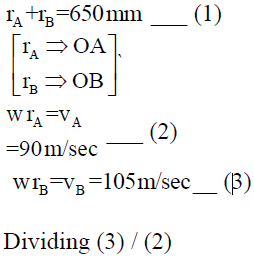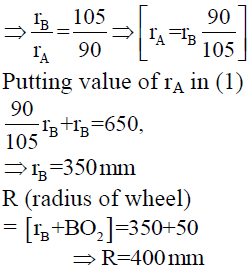Theory of Machines - Question 22

In the figure given the link 2 rotates at an angular velocity of 2 rad/sec. what is the magnitude of corioli’s, acceleration experienced by link 4 at given instance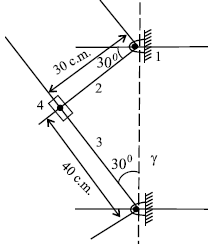Detailed Solution for Theory of Machines - Question 22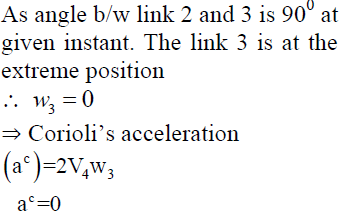*Answer can only contain numeric values
Theory of Machines - Question 23

For a mechanism consisting of 14 links, to have one degree of freedom with all revolute pairs. The no. of revolute pairs is ________

(Important - Enter only the numerical value in the answer)

Detailed Solution for Theory of Machines - Question 23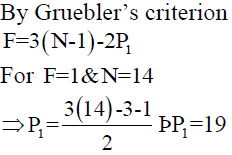*Answer can only contain numeric values
Theory of Machines - Question 24

For the mechanism shown Angular velocity of link AO2 at this instant is _____ rad/sec

[Use instantaneous centre Method]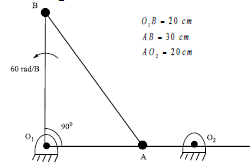(Important - Enter only the numerical value in the answer)

Detailed Solution for Theory of Machines - Question 24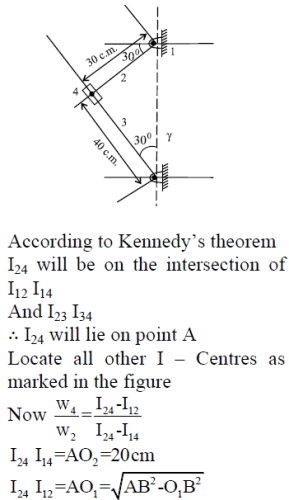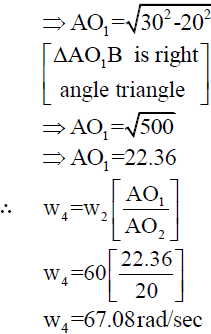*Answer can only contain numeric values
Theory of Machines - Question 25

Two disc 1 and 2 are arranged as shown in the figure are pined at centres O1 and O2 and have radii r1 =4m and r2 =8m respectively. If disc 1 is rotating at 600rpm. Difference between the velocities at point A and B is ________ m/sec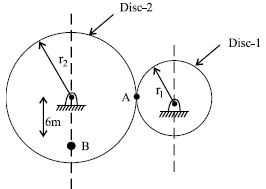(Important - Enter only the numerical value in the answer)

Detailed Solution for Theory of Machines - Question 25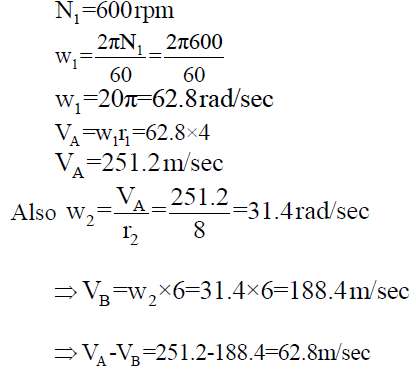## GATE Mechanical (ME) 2023 Mock Test Series

27 docs|243 tests
Information about Theory of Machines Page
In this test you can find the Exam questions for Theory of Machines solved & explained in the simplest way possible. Besides giving Questions and answers for Theory of Machines, EduRev gives you an ample number of Online tests for practice

## GATE Mechanical (ME) 2023 Mock Test Series

27 docs|243 tests(Scan QR code)